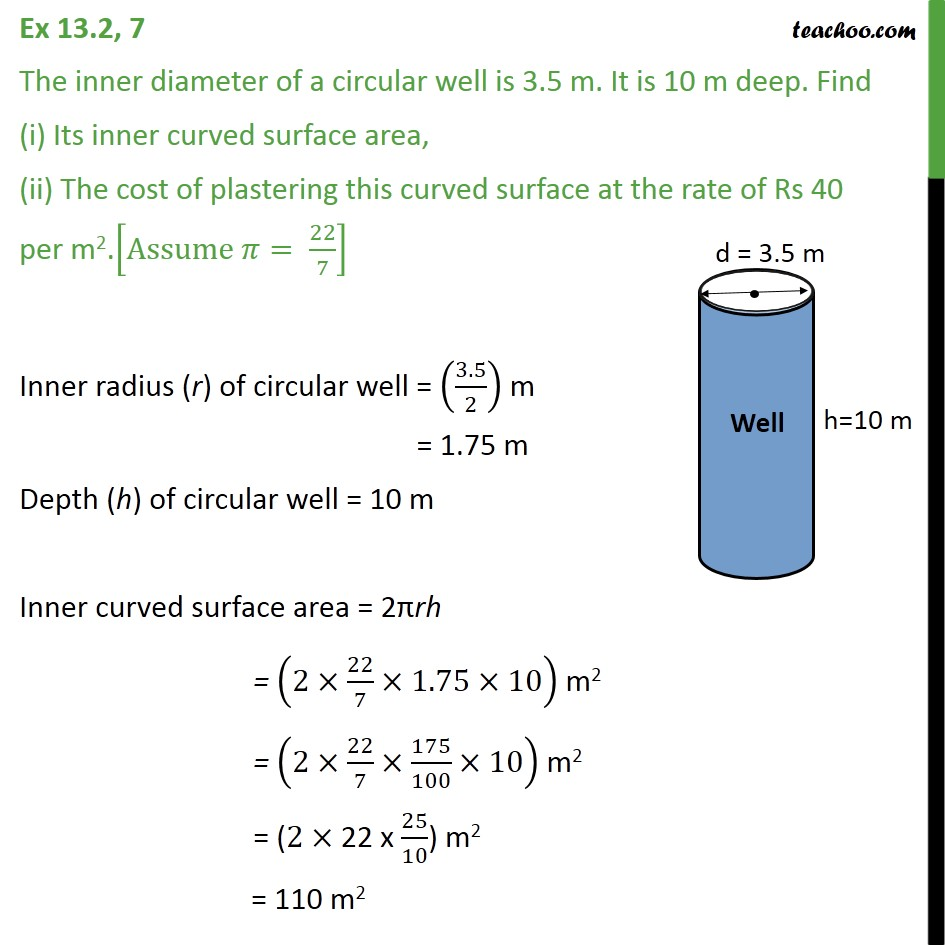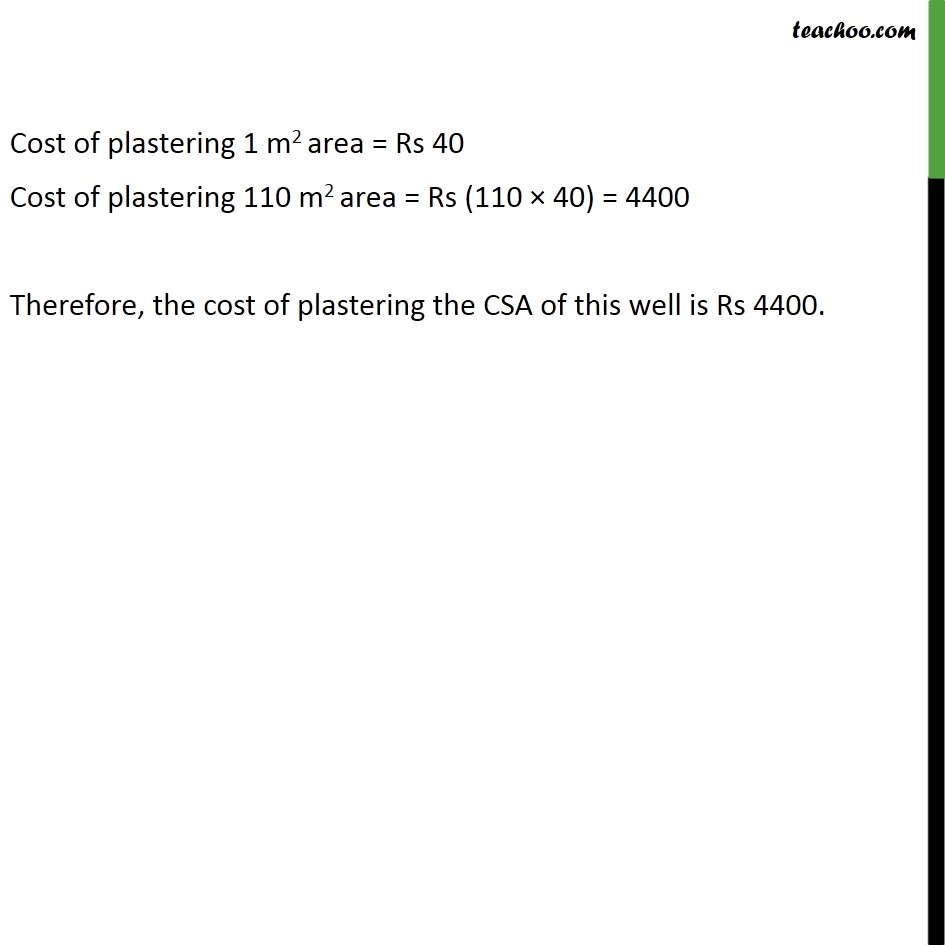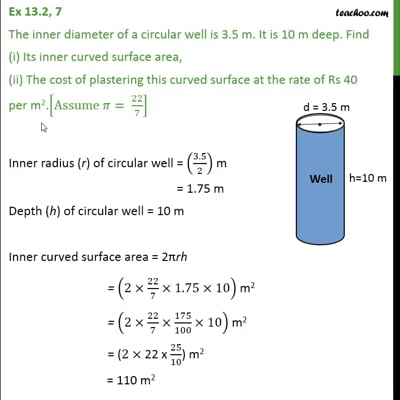Ex 13.2

Chapter 13 Class 9 Surface Areas and Volumes
Serial order wiseThis video is only available for Teachoo black users

Get live Maths 1-on-1 Classs - Class 6 to 12

### Transcript

Ex 13.2, 7 The inner diameter of a circular well is 3.5 m. It is 10 m deep. Find (i) Its inner curved surface area, (ii) The cost of plastering this curved surface at the rate of Rs 40 per m2.[Assume 𝜋= 22/7] Inner radius (r) of circular well = (3.5/2) m = 1.75 m Depth (h) of circular well = 10 m Inner curved surface area = 2πrh = (2×22/7×1.75×10) m2 = (2×22/7×175/100×10) m2 = (2× 22 x 25/10) m2 = 110 m2 Cost of plastering 1 m2 area = Rs 40 Cost of plastering 110 m2 area = Rs (110 × 40) = 4400 Therefore, the cost of plastering the CSA of this well is Rs 4400.